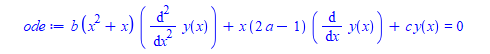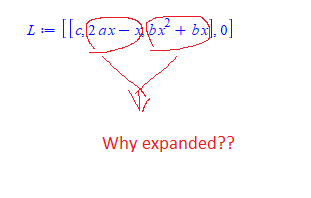## How to keep DEtools:-convertAlg from expanding coe...

It will be great if  DEtools:-convertAlg  which is designed to find coefficients of a linear ode, not to expand the coefficients it finds.

This caused my patterns to fail, as after putting the coefficients in a certain form, and then calling DEtools:-convertAlg later on somewhere else in the program, the patterns no longer worked due to this unexpected expansion.

It will be better if DEtools:-convertAlg returns the coefficients it find as is and not expand them. I could not find an option to use for this. Here is an example.

```restart;
ode:=b*(x+x^2)*diff(y(x),x\$2)+x*(2*a - 1)*diff(y(x),x)+c*y(x)=0;
```After the call the coefficients become

`L:=DEtools:-convertAlg(ode,y(x))`You might ask that one can easily "fix" this afterwords. Sure. I can use collect on x. But the point is that in general, it might not be clear what to collect on or what the changes that were made are.

In principle, it is better to keep the coefficients in same form as they were in the ode so that the ode do not change form.

Maple 2022.1 on windows.

## How do I read email messages in Maple ?...

```For example my friend emailed me.
`His email address is oneman@gmail.com, the email content is HELLO.`
`How does Maple print HELLO to the screen so I can see it?`
`Thanks for your help!`
 >